# How to build a concrete staircase

The construction of concrete steps requires full knowledge of concrete mix, concrete pouring techniques and the use of appropriate tools, such as: hammers, power drills, building scales...etc. If you are just getting started on your first concrete project, you may want to practice doing simpler work like pouring and leveling concrete on the floor. This type of work is heavy industry, so you may want to check with your doctor first if you are not confident in your physical ability to perform such strenuous tasks. If you are up for the challenge, with a little planning and attention to detail you will be able to add the concrete steps to your building yourself; At a fraction of the cost much lower than that which the contractor would require.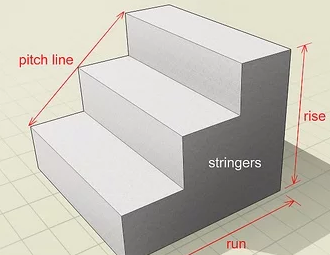1
Learn vocabulary related to building stairs. Each rung of the ladder consists of two main parts: the “upright” which is the vertical part of the rung, and the “sleeper” which is the part you step on; Other important terms to know when building stairs include:

The slope line whose edge measures the angle your steps make from the edge of the lower step to the edge of the higher step.
The height is the total height of your ladder from its base to its highest rung.
Handrail which is the side pillars for both sides of the staircase. You will use your side measurements to make your concrete molds.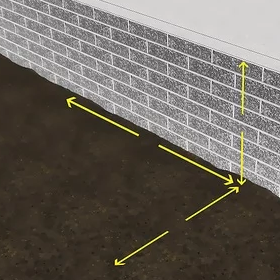2
Measure the dimensions of your ladder. First you have to know the total height of the ladder, which can be calculated by measuring the total height from the established base to the highest floor level you want to reach, then:

Measure the rung by finding the distance between the farthest front and the farthest back of the ladder.
Find the amplitude of the steps by measuring the distance between the rightmost and leftmost boundaries of the planned steps.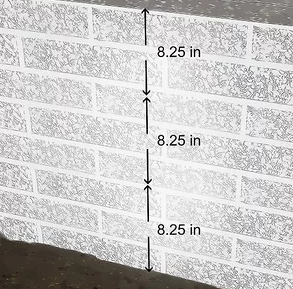3
Calculate the number of degrees you need. You can do this by dividing the (total height) of the ladder by the height of the post (the height of each rung). The building code in Egypt limits the average slate to 15 centimeters, refer to the Egyptian building code or consult an engineer who knows the matter.

If the division is a fraction, be sure to round the whole number.
Note that if your ladder is built so that the top of the sleepers is one degree lower than the floor you want to reach then the number of (sleepers) needed will be one less than the number of uprights.
There are many sites on the Internet that allow you to calculate scores to simplify the process. You should be able to find it with a general internet search for "stair calculators". These calculators help you determine the exact specifications of your grade, including the number of decks, the height of each post, the length of the auxiliary longitudinal ribs, the slope line, and so on.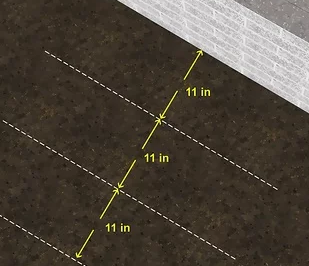4
Select the height and depth of the sleeper. We can be flexible in determining the depth of each sleeper, but it must be on average and common in Egypt 30 centimeters, so that it fits both large and small feet. As for finding the height of each list you must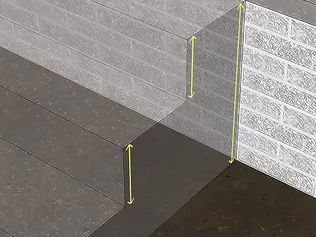5
Calculate the height of the stairs. Divide the total height of the entire ladder by the number of steps. In general, you should round the number you get for the step height to the nearest 1.5mm.